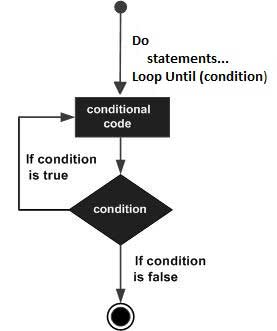# VB.Net - Do Loop

It repeats the enclosed block of statements while a Boolean condition is True or until the condition becomes True. It could be terminated at any time with the Exit Do statement.

The syntax for this loop construct is −

```Do { While | Until } condition
[ statements ]
[ Continue Do ]
[ statements ]
[ Exit Do ]
[ statements ]
Loop
-or-
Do
[ statements ]
[ Continue Do ]
[ statements ]
[ Exit Do ]
[ statements ]
Loop { While | Until } condition
```

## Flow Diagram## Example

```Module loops
Sub Main()
' local variable definition
Dim a As Integer = 10
'do loop execution
Do
Console.WriteLine("value of a: {0}", a)
a = a + 1
Loop While (a < 20)
End Sub
End Module
```

When the above code is compiled and executed, it produces the following result −

```value of a: 10
value of a: 11
value of a: 12
value of a: 13
value of a: 14
value of a: 15
value of a: 16
value of a: 17
value of a: 18
value of a: 19
```

The program would behave in same way, if you use an Until statement, instead of While −

```Module loops
Sub Main()
' local variable definition
Dim a As Integer = 10
'do loop execution

Do
Console.WriteLine("value of a: {0}", a)
a = a + 1
Loop Until (a = 20)
End Sub
End Module
```

When the above code is compiled and executed, it produces the following result −

```value of a: 10
value of a: 11
value of a: 12
value of a: 13
value of a: 14
value of a: 15
value of a: 16
value of a: 17
value of a: 18
value of a: 19
```
vb.net_loops.htm